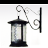# ArcGIS 10.1 Tabulate Intersection: confused

4000
0
10-31-2012 12:19 AMRegular Contributor III
Hi folks,

I have been testing using the new tool introduced in ArcGIS 10.1 called Tabulate Intersection. However, I am not getting the results I expect. Let me give you the details.

I have two polygon feature classes, first - represents administrative area units (say counties), second - the zone of interest. Each county polygon has a certain integer attribute field where a statistics value stored. What I am trying to achieve is to get to know what part of the county is located within the zone of interest (purely geometrical intersection) and then based on the percentage calculate the statistics value that will be allocated to the zone of interest. So, say in the zone of interest (red polygon), only 10% of the area of the county with the attribute value of 223 is located. This means, a smaller intersecting polygon that represents shared area between the county and the zone of interest gets the value of 22.3. Please refer to the figure attached.

Afterwards, I want to go through all other counties that intersect the zone of interest and calculate the value proportionally as outlined above. Then those intersecting polygons with newly allocated values are dissolved based on the zone of interest ID and their attribute value are summed.

All of this works fine in a newly built GP model where I run the Intersect first, then join the original counties to get their Shape_Area for area percentage calculation, then calculate the statistics value with the regards to the area percentage, then Dissolve those intersecting polygons and I get the results expected.

However, I was thinking to test the Tabulate Intersection tool which would give the table with the percentage value for every(?) intersecting polygon, so I could join the output table to the intersected polygons layer. So, when I run the tool, I get a smaller number of rows in the output table comparing to the output layer when I run Intersect for the zone of interest layer and counties layer (174 to 188). Yet I thought it would give me back a table where each row represents an intersecting polygon.

Questions:

1) Did I misunderstand how the Tabulate Intersection tool works? Should it give back the same number of rows as when running the Intersect?
2) Is there any other more efficient way to solve the problem described? Ideas for considerations are welcome 🙂

Thank you,
Alex
Tags (3)
0 Replies